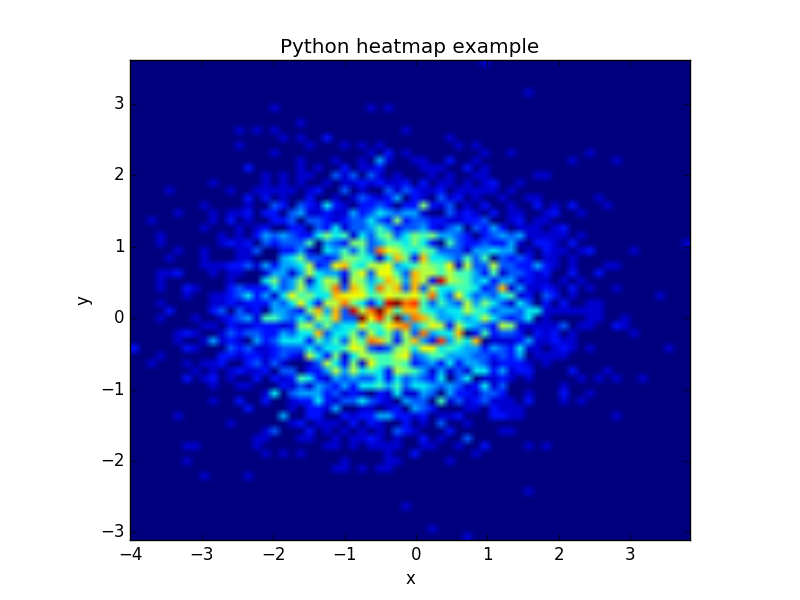Generate heatmap in Matplotlib

A heatmap can be created using Matplotlib and numpy.

Related courses
If you want to learn more on data visualization, these courses are good:

Heatmap example

The histogram2d function can be used to generate a heatmap.

We create some random data arrays (x,y) to use in the program. We set bins to 64, the resulting heatmap will be 64x64. If you want another size change the number of bins.

Result:Matplotlib heatmap

The datapoints in this example are totally random and generated using np.random.randn()

One thought on “Generate heatmap in Matplotlib”

1.- December 21, 2017

It’s not obvious from the example given, but this will flip the image. to correct that change the following line :
plt.imshow(heatmap, extent=extent)
to:
plt.imshow(heatmap, extent=extent, origin=’lower’)